VIII. Hot rods

## Linear Methods of Applied Mathematics Evans M. Harrell II and James V. Herod

(Some remarks for the instructor).

version of 7 Dec 2000

If you wish to print a nicely formatted version of this chapter, you may download the rtf file, which will be interpreted and opened by Microsoft Word or the pdf file, which will be interpreted and opened by Adobe Acrobat Reader.

Some of the calculations of this chapter are available in a Mathematica notebook or in a Maple worksheet.

## VIII. The Mathematics of Hot Rods

In many ways the simplest of our three model partial differential equations is the heat equation, also known as the diffusion equation,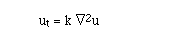(HE-3D)

or, specializing for now to one dimension,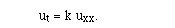(HE)

The heat equation is the simplest model of a parabolic partial differential equation. A physical derivation of the heat equation is given in an appendix; the constant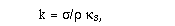whereis the thermal conductivity,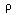is the density, and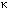s is the specific heat capacity of a homogeneous substance. Ordinary substances have values of k ranging from about 5 to 9000 cm2/hr (see table).

We shall solve the heat equation with the method of separation of variables, as we did for the wave equation. The solutions will be quite similar, and you may find it instructive to compare with what we did in chapter VI. We begin with the one-space-dimensional heat equation (HE), which can be written with the help of a heat operator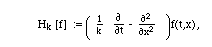The heat equation is the equation for the kernel of this operator. This equation describes the temperature u(t,x) in a long, thin rod along the x-axis, if the rod is wrapped along its length with a perfect insulator. The heat equation is a linear, homogeneous equation, so, just as for the wave equation, the superposition principle holds; if we can find some solutions u1, ..., uk, then any linear combination of these solutions is again a solution.

With the method of separation of variables, we try to find solutions of a special form,

u = T(t) X(x).

As before, boundary and initial conditions will be important, and arise from the physical situation we wish to study. The end points of the rod may be in thermal contact with ice water, which will ensure that we have homogeneous Dirichlet boundary conditions (DBC)

u(t,a) = u(t,b) = 0.

(Actually, stirred wet crushed ice would guarantee these boundary conditions in the laboratory better than ice water.)

The fact that these boundary conditions are homogeneous (something equals 0) is going to be useful in our analysis, so you may wonder if something goes wrong if we use a different temperature scale or hold the ends at a different fixed temperature T different from 0. Fortunately, we only need to redefine u by a constant to have these same boundary conditions. Since Hk[T] = 0, if

v(t,x) := u(t,x) - T,           (8.1)

then

Hk[v] = Hk[u] = 0.

By this simple change, the problem with some other fixed temperature at the ends is converted into out standard problem with homogeneous boundary conditions of the Dirichlet type.

Neumann boundary conditions (NBC),

ux(t,a) = ux(t,b) = 0,

correspond physically to the situation when the ends of the rod are also insulated, since the heat flow through either end of the rod is proportional to the temperature gradient at that end. If there is no heat flow, the gradient must be 0 (recall the derivation).

We begin by considering the case of Dirichlet boundary conditions, and suppose that the rod has length 1, with a = 0 and b = 1. We shall solve this problem with the method of separation of variables, by looking for special solutions analogous to normal modes for the wave equation. (A live version of this deivation is available in the Mathematica notebook or the Maple worksheet for this chapter. It is also instructive to compare with chapter VI.) Ignoring the initial conditions for the time being, we begin by seeking product solutions, u(t,x) = T(t) X(x) to the heat equation ( HE), which also satisfy the homogeneous boundary conditions.

The efficient way to proceed is to divide the wave equation by the product solution: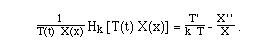If u solves the heat equation, this expression must be zero. Thus

T'/(k T) = X''/X.

Since the left side is independent of x and the right side is independent of t, both sides must in fact a constant. We don't know its value yet, so let us call it -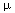. (It will turn out to be negative.)

Thus

-X''(x) =X(x)           (8.2)

This is precisely the same eigenvalue equation as in chapter VI, for the wave equation with (DBC). We know that the eigenfunctions and eigenvalues are:

X(x) := sin(n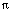x),

u = (n)2.

The other part of the partial differential equation which has been separated differs from what we got with the wave equation:

T'(t) = -n k T(t)           (8.3))

This is a simple first-order equation, the solutions of which are exponential functions,

T[t] = C exp(-n k t).

The eigenvalue problem (8.2) fixed the possibilities forn, leaving us with normal modes of the form

cn exp(- n22 t) sin(nx), n = 1, ...

With DBC at a and b, b-a = L, we would get:

cn exp(- n22 k t) sin(n(x-a)/L), n = 1,...;

the easiest way to see this is to change variables to x' = (x-a)/L. In passing, we note that we could have set k=1 throughout the derivation of the normal modes, and then changed variables to t' = k t at the end.

Since the heat equation is linear, we can make linear combinations of the solutions we have found and thereby produce new solutions. The most general solution to the heat equation that we get in thisway is:

Sum{n=1}{ cn exp(- n22 t) sin(nx)}.          (8.4)

At this stage it may not be completely clear that this is the absolutely most general solution, but the Fourier sine series will guarantee that this can indeed describe the solution to the heat equation with an arbitrary square-integrable initial condition.

The initial conditions appropriate to the heat equation are somewhat different from what we had for the wave equation, again because the time variable enters differently. The heat equation is first-order in time, so we need only one initial condition, the initial temperature distribution in the rod,

u(t,x) = f(x).           (IC)

There is no need to look at ut at t=0. Physically, this would be inappropriate, because our experience teaches us that the temperature changes only in response to the initial temperature, not to some unmeasurable effect of the rate of change of the initial temperature. If this were not the case, cookbooks would be nearly impossible. Fortunately, the initial conditions to bake a cake are, typically, batter at room temperature, and if we have identical batter in identical pans at room temperature, we need to bake the cake for identical lengths of time. No other physical initial condition is necessary.

Model Problem VIII.1. Find the future temperature within a rod of length 1, k=1, insulated along its length, and with ends in thermal contact with ice water, if at time 0, the rod is at a uniform temperature u(0,x) = 50.

Solution. (See Mathematica notebook or Maple worksheet for the calculations.)

At time t=0, the general solution becomes a Fourier sine series,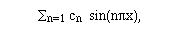so the coefficients cn are determined as the Fourier sine series coefficients of the initial function, f(x) = 50. We know the formula for the Fourier sine coefficients, (2.15), so we simply calculate the integral to get: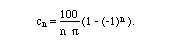This is 0 when n is even and otherwise 200/(n). Our solution to the heat equation is thus: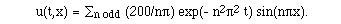Suppose now that the rod is insulated not only along its length but also on its ends. Then u(t,0) and u(t,L) are not specified, but instead we have: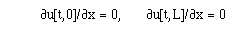(NBC)

Model Problem VIII.2. Set up the general solution of the insulated rod with Neumann BC., with the simplifications k = L = 1.

Solution.

This is very similar to the analysis in chapter VI, and the eigenfunctions are the basis functions of the Fourier cosine series,

{1, cos(nx)}.

The normal modes are

cn exp(- n22 t) cos(nx), n = 0, 1, ...

and the general solution is: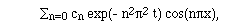(8.5)

Notice that after a long time, all of the terms of this series will become negligible except the one corresponding to n=0. Remembering that c0 is nothing other than the average temperature, we see that if the rod is entirely insulated, then after a long time the temperature has settled down to equilibrium at the one temperature which is consistent with conservation of energy.

Another way to see the same effect is as follows. (I shall do the calculation in three dimensions, since it is not much harder than in one dimension.) In the derivation of the heat equation, we reason that the rate of change of heat content is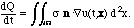If the object is insulated, then ndot (Grad u) = 0. (This higher-dimensional Neumann condition is what simplifies to u_x = 0 in one space dimension.) It follows that the total heat content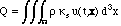remains constant. For a homogeneous material, this is just a multiple of the average temperature.

Something more subtle happens in the case of DBC, where energy is lost (or gained) through the ends of the rod. In that case, the general solution (8.4) ensures that not only will the temperature settle down to an equilibrium u(t,x) -> 0 as x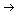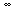, but it usually will do so with a particular temperature profile. Assuming that c1 is not 0, we find that

exp(2t) u(t,x) = c1 + r(t,x),

where the remainder terms in

r(t,x) = Sum{n=2} cn exp(- (n2-1)2 t) sin(nx)

all vanish rapidly as t->infinity. As can be seen from the calculations in the Mathematica notebook, the temperature profile of the rod rapidly approaches that of a simple sine function, times a time-varying factor tending to 0.

Model Problem VIII.3. Suppose now that we have non-homogeneous boundary conditions, such as what we would get if the end of the rod at x=0 is held at temperature 100 and the end at x=1 at temperature 0. Find the general solution to the heat equation with k=1.

Solution.

We can no longer convert this problem to the usual one by the simple trick (8.1). A better idea is to think about what should happen at equilibrium in this problem. At equilibrium, ut = 0, so the temperature will depend on space alone according to

u(eq)xx = 0.          (8.6)

In this one-dimensional situation, this means that u(eq)(x) = c1 x + c2 - the general solution to (8.6). With the given boundary conditions, we can easily solve for the constants, and find that

u(eq)(x) = 100 - 100 x.

Since the heat equation is linear we can adjust the solution to obtain our familiar boundary conditions by subtracting the equilibrium, letting

v(t,x) := u(t,x) - ueq(x).

It is easy to see that

Hk[u - ueq] = 0,

and the general solution is thus

u(t,x) = 100 - 100 x + Sum{n=1} cn exp(- n22 t) sin(nx)

The properties of a solution to the heat equation are quite different from those of the wave equation. Instead of wave motion and a tolerance for discontinuity, we will find

1. Equilibration - after a long time, the solution becomes independent of time.

2. Intolerance for extremes and discontinuity. Even if the solution starts off as quite a wild function f(x), it will instantaneously become a smooth function, which can be differentiated arbitrarily often.

We investigated equilibration above, but have not yet seen how the heat equation enforces smoothness. One aspect of this phenomenon can be seen from the general solution, say with DBC, (8.4). We learned in chapters II-III that a function can behave rather terribly and still be the sum of a Fourier series, where the squares of the coefficients must tend to 0 as n, owing to the Parseval formula (3.4). Because of this, suppose that all we know about the coefficients is that they are bounded by some tremendous number B. Now, if we could say that we are safe in neglecting the terms in a Fourier series beyond some finite point, n > N, then we would be left with a finite sum of sines or cosines, and that would clearly be differentiable as often as we would like. We also learned in chapter V that we can safely differentiate Fourier series, provided that the differentiated series makes sense.

With this in mind, let's differentiate our general solution, say, 900 times with respect to x. It is not hard to see from (8.4) that we get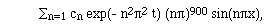(8.7)

where all we know about the cn is that they are bounded by B. (We would have some minuses or cosines if we differentiated a number of times not divisible by 4.) If t=0, we have amplified all the coefficients, but if t > 0, then for large n, the exponential goes to zero faster than any power of n, and the series (8.7) converges uniformly. This happens no matter how small t is, and shows that the 900th x-derivative of u exists as a continuous function (a theorem from advanced calculus states that a uniform limit of continuous functions is continuous).

A careful analysis suggested by this argument proves that even if the initial condition is extremely irregular, a solution of the heat equation instantly becomes smooth. A precise definition of what we mean by "smooth" is as follows.

Definition VIII.4. A function is smooth, or C, if it can be differentiated arbitrarily often.

Theorem VIII.5. Let u solve the heat equation on an interval a < x < b, and t > 0, with initial condition u(0,x) = f(x) square integrable and either Dirichlet boundary conditions or Neumann boundary conditions. Then for all t > 0, u(t,x) is smooth.

Later we shall consider the heat equation on higher dimensional regions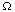. The same theorem holds for all x in the interior of. Also, other standard kinds of boundary conditions can be allowed in this theorem.

Another important property of the heat equation is the maximum principle.

Theorem VIII.6. Let u solve the heat equation. If we consider any finite space-time region, such as a rectangle a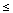xb, ctd, then the greatest value of the temperature occurs on the boundary of the region, in this case when x = a or b or when t = c or d. In other words, a hot spot cannot be spontaneously generated in the interior of the material. The same is true of the minimum, a potential cold spot - the minimum always occurs on the boundary.

As with the smoothing theorem, the maximum principle also applies to higher-dimensional regions.

To prove the theorem, let us first consider the heat equation with a heat sink, which absorbs energy. The equation describing this would be

ut = uxx - Q(t,x),                     (8.8)

where Q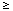0. For now, suppose that Q is strictly positive, so Q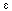> 0 throughout the rod. Suppose that there is a hot spot, i.e., a local maximum, at (t0,x0), in the interior of the space-time region. By basic calculus, the space-time gradient of u must be 0 there (we know that the solution is differentiable), that is, ut(t0,x0) = ux(t0,x0) = 0. If the point is really a maximum, then uxx(t0,x0)0. But this is a contradiction, since

uxx = ut + Q> 0.
This shows that there can be no local maximum in the interior of the region, so the only possibility is for the maximum to be on the boundary.

Now suppose that there is no sink, so we have a solution of the heat equation with no sinks or sources,

ut = uxx,
and that there is a point (t0,x0) where the temperature exceeds that on the boundary of a finite region. To be specific, suppose that we replace u with the function

v(t,x) := u(t,x) +exp(x),

whereis a small but positive quantity, so small that the maximum value of v is also attained in the interior - this is possible by the intermediate value theorem of calculus, which applies to continuous functions. What equation does v solve?

vt = ut,

while

vxx = uxx +exp(x) = ut +exp(x) = vt +exp(x).

Solving for vt, we have

vt = vxx - Q,

where Q =exp(x) is strictly positive. We already considered this case, and found that it cannot happen. The transformation shows that it cannot happen for the basic heat equation, either.

The statement about the minimum follows because -u solves the heat equation whenever u does.

```                                                                                                                                              QED
```

Exercises VIII

1. Solve the heat equation with DBC at 0 and 1 and:
f(x) = |x-1/2|.
2. Solve the heat equation with NBC at 0 and 1 and:
f(x) = sin(x)
3. Put DBC at x=0 and NBC at x=L. Separate variables, solve the eigenvalue problem for X[x], and write down a general solution.
4. Solve the heat equation with IC:
f(x) = 1
and
1. DBC at x=0 and x=1
2. NBC at x=0 and x=1
3. DBC at x=0 and NBC at x=1
Plot the solutions and compare.
5. Find some initial conditions for which a unit rod with DBC will not have an asymptotic temperature profile with spatial dependence sin(x), and use the computer to study the approach to equilibrium in this case.
6. Solve the initial-boundary value problem:
ut = uxx
u(t,0) = 50, u(t,2) = 100
u(0,x) = 20 x
7. Solve the initial-boundary value problem
ut = uxx + 1
u(t,0) = 100, u(t,2) = 100
u(0,x) = 20 x
Hint: subtract something to eliminate the non-homogeneity in the PDE as well as in the BC.
8. Find the general solution by separation of variables of the boundary-value problems
1. ut = 4 uxx + u
u(0,t) = u(2,t) = 0
2. ut = 4 uxx + ux
u(0,t) = u(2,t) = 0
3. ut = 4 uxx + u + ux
u(0,t) = u(2,t) = 0
9. Use the ratio test and l'Hôpital's rule of advanced calculus to show that the series (8.7) converges uniformly.
10. It is possible for a rod to be in equilibrium at a constant temperature, and in that case it attains its maximum, and only, temperature in the interior. Explain why this state of affairs is consistent with the maximum principle and with its proof. Prove the maximum principle with 2 or 3 spatial variables, using the same argument.
11. Does the maximum principle hold for ut = uxx + Q(t,x), Q > 0? Either give a proof or a counterexample. Interpret physically.